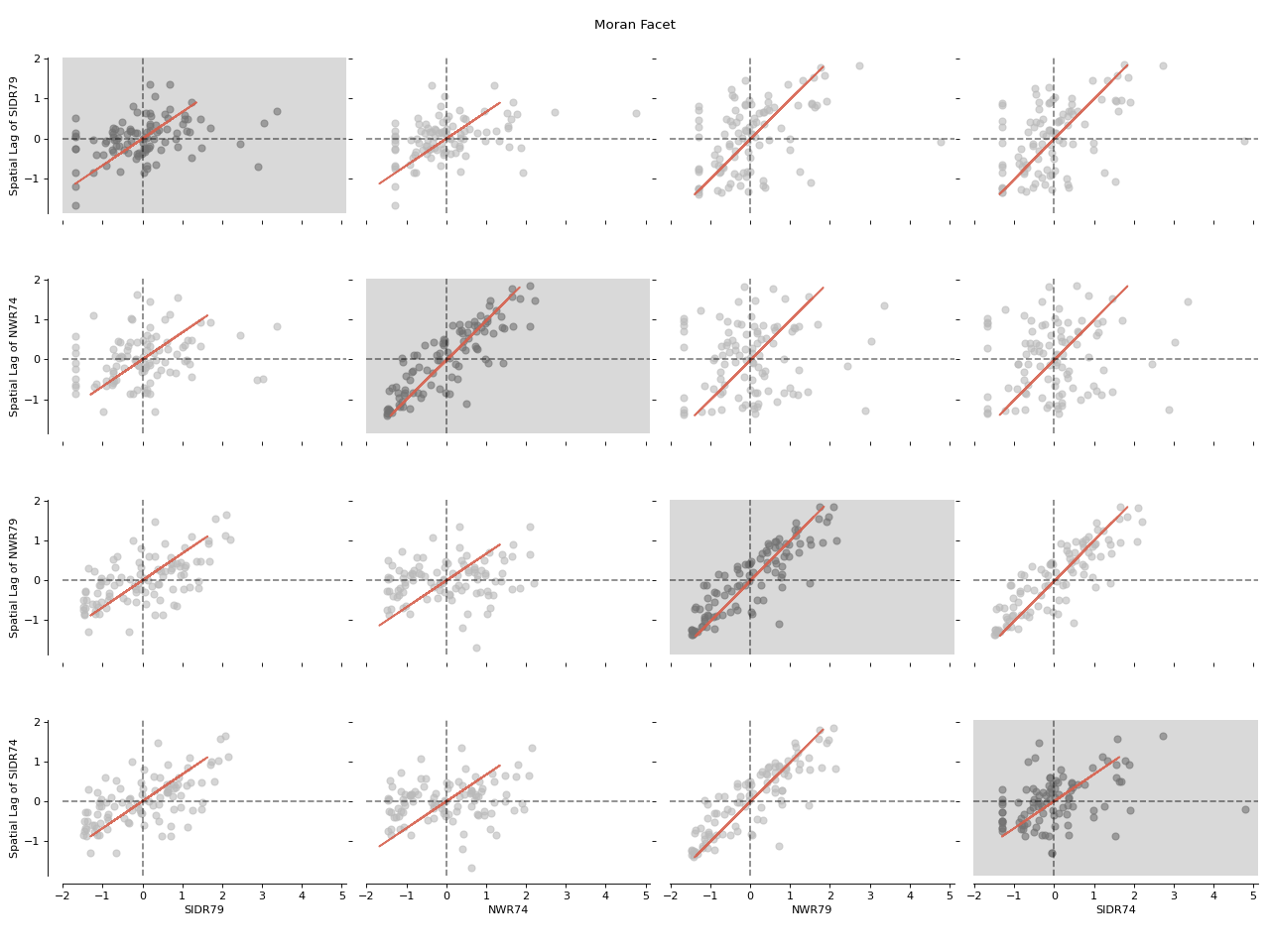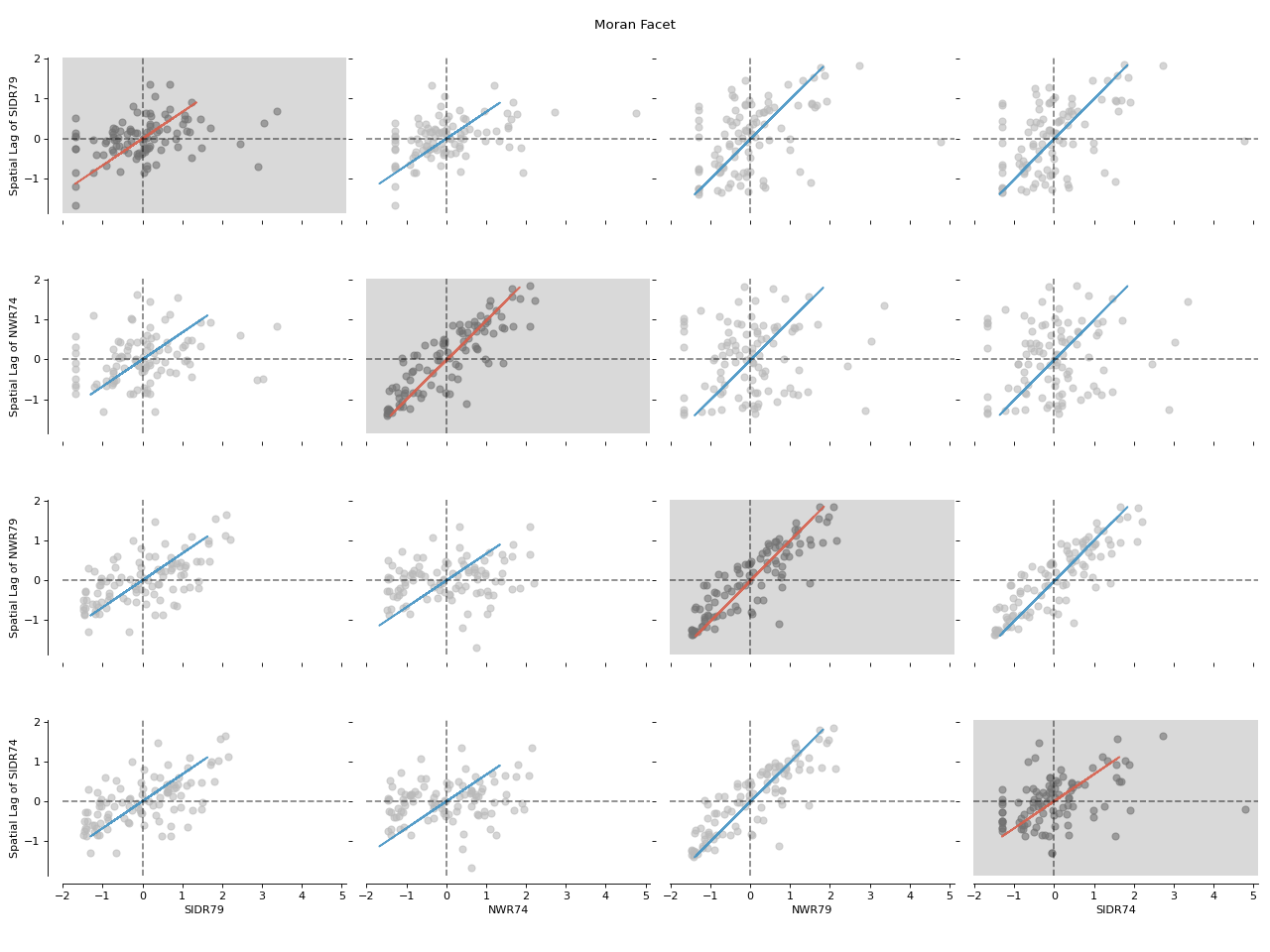splot.esda.moran_facet¶

splot.esda.moran_facet(moran_matrix, figsize=(16, 12), scatter_bv_kwds=None, fitline_bv_kwds=None, scatter_glob_kwds={'color': '#737373'}, fitline_glob_kwds=None)[source]

Moran Facet visualization. Includes BV Morans and Global Morans on the diagonal.

Parameters
moran_matrixesda.moran.Moran_BV_matrix instance

Dictionary of Moran_BV objects

figsizetuple, optional

W, h of figure. Default =(16,12)

scatter_bv_kwdskeyword arguments, optional

Keywords used for creating and designing the scatter points of off-diagonal Moran_BV plots. Default =None.

fitline_bv_kwdskeyword arguments, optional

Keywords used for creating and designing the moran fitline of off-diagonal Moran_BV plots. Default =None.

scatter_glob_kwdskeyword arguments, optional

Keywords used for creating and designing the scatter points of diagonal Moran plots. Default =None.

fitline_glob_kwdskeyword arguments, optional

Keywords used for creating and designing the moran fitline of diagonal Moran plots. Default =None.

Returns
figMatplotlib Figure instance

Bivariate Moran Local scatterplot figure

axarrmatplotlib Axes instance

Axes in which the figure is plotted

Examples

Imports

>>> import matplotlib.pyplot as plt
>>> import libpysal as lp
>>> import numpy as np
>>> import geopandas as gpd
>>> from esda.moran import Moran_BV_matrix
>>> from splot.esda import moran_facet

Load data and calculate Moran Local statistics

>>> varnames = ['SIDR74',  'SIDR79',  'NWR74',  'NWR79']
>>> vars = [np.array(f[var]) for var in varnames]
>>> moran_matrix = Moran_BV_matrix(vars,  w,  varnames = varnames)

Plot

>>> fig, axarr = moran_facet(moran_matrix)
>>> plt.show()Customize plot

>>> fig, axarr = moran_facet(moran_matrix,
...                          fitline_bv_kwds=dict(color='#4393c3'))
>>> plt.show()

(png, hires.png, pdf)